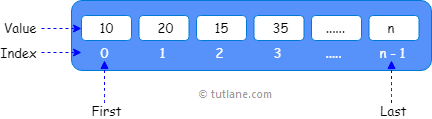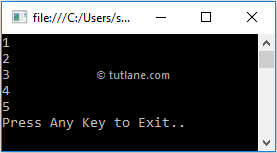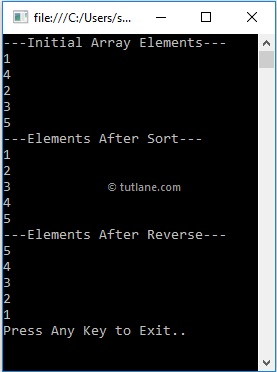# Visual Basic (VB) Arrays

In visual basic, Arrays are useful to store multiple elements of the same data type at contiguous memory locations and arrays will allow us to store the fixed number of elements sequentially based on the predefined number of items.

In the previous chapter, we learned about variables in visual basic, which will help us to hold a single value like Dim id As Integer =10. In case, if we want to hold more than one value of the same data type, then the arrays came into the picture in visual basic to solve this problem.

An array can start storing the values from index 0. Suppose if we have an array with n elements, then it will start storing the elements from index 0 to n-1.

Following is the pictorial representation of storing the multiple values of the same type in a visual basic array data structure.If you observe the above diagram, we are storing the values in an array starting from index 0 and it will continue to store the values based on the defined number of elements.

## Visual Basic Arrays Declaration

In visual basic, Arrays can be declared by specifying the type of elements followed by the brackets () like as shown below.

Dim array_name As [Data_Type]();

Here, array_name represents the name of an array and Data_type will represent the data type of elements to store in an array.

For example, the following are the different ways of declaring an array with different data types in a visual basic programming language.

' Store only int values

Dim numbers As Integer()

' Store only string values

Dim names As String()

' Store only double values

Dim ranges As Double()

If you observe the above examples, we declared arrays with required data types based on our requirements.

In visual basic, array elements can be of any type and by default, the values of numeric array elements are set to zero and the reference elements are set to null.

## Visual Basic Arrays Initialization

In visual basic, Arrays can be initialized by creating an instance of an array with `New` keyword. By using the `New` keyword, we can declare and initialize an array at the same time based on our requirements.

Following are the different ways of declaring and initializing array elements by using `New` keyword in a visual basic programming language.

' Declaring and Initializing an array with size of 5

Dim array As Integer() = New Integer(4) {}

' Defining and assigning an elements at the same time

Dim array2 As Integer() = New Integer(4) {1, 2, 3, 4, 5}

' Initialize with 5 elements will indicates the size of an array

Dim array3 As Integer() = New Integer() {1, 2, 3, 4, 5}

' Another way to initialize an array without size

Dim array4 As Integer() = {1, 2, 3, 4, 5}

' Declare an array without initialization

Dim array5 As Integer()

array5 = New Integer() {1, 2, 3, 4, 5}

If you observe the above examples, in the first statement we declared and initialized an integer array with the size of 5 to allow an array to store 5 integer values and the array can contain elements from array to array.

In the second statement, we declared and initialized an array same like the first statement but also assigned a value to each index followed by curly brackets { }.

In third and fourth statements, while declaration we initialized an array with values, but without specifying any size. Here, the size of an array can be determined by the number of elements so the size initializer is not required if we are assigning elements during the initialization.

In visual basic, we can declare an array variable without initialization, but we must use the `New` keyword to assign an array to the variable.

In the fifth statement, we declared an array without initialization and we used `New` keyword to assign array values to the variable.

In visual basic after an array declaration, we can initialize array elements using index values. Following is an example of declaring and initializing array elements using individual index values in visual basic.

int[] array = new int;

array = 1;

array = 2;

array = 3;

array = 4;

array = 5;

If you observe the above example, we initialized array elements separately by using index values.

## Visual Basic Accessing an Array Elements

In visual basic, we can access array elements by using for loop or foreach loop or with particular index numbers.

Following is the code snippet of accessing array elements by using particular index numbers.

Dim array As Integer() = New Integer(4) {1, 2, 3, 4, 5}

Dim a As Integer = array(1)

Dim b As Integer = array(4)

If you observe the above code, we are trying to access array elements using index values in visual basic.

Following is the example of declaring, initializing and accessing array elements with particular index numbers in a visual basic programming language.

Module Module1

Sub Main()

Dim array As Integer() = New Integer(4) {1, 2, 3, 4, 5}

Console.WriteLine(array(0))

Console.WriteLine(array(1))

Console.WriteLine(array(2))

Console.WriteLine(array(3))

Console.WriteLine(array(4))

Console.WriteLine("Press Enter Key to Exit..")

End Sub

End Module

If you observe the above example, we declared and initialized an array with 5 elements and we are accessing array elements using index values.

When we execute the above visual basic program, we will get the result as shown below.If you observe the above result, we are able to access array elements using index numbers based on our requirements.

## Visual Basic Access Array Elements with For Loop

In visual basic, by using for loop we can iterate through array elements and access the values of an array with length property.

Following is the example of accessing array elements using for loop in a visual basic programming language.

Module Module1

Sub Main()

Dim array As Integer() = New Integer(4) {1, 2, 3, 4, 5}

For i As Integer = 0 To array.Length - 1

Console.WriteLine(array(i))

Next

Console.WriteLine("Press Enter Key to Exit..")

End Sub

End Module

If you observe the above example, we are looping through array elements with for loop to access array elements based on our requirements.

When we execute the above visual basic program, we will get the result as shown below.If you observe the above result, we are able to loop through the elements of an array with for loop and able to print array values based on our requirements.

## Visual Basic Access Array Elements with Foreach Loop

In visual basic, same as for loop we can use For Each loop to iterate through array elements and access the values of an array based on our requirements.

Following is the example of accessing array elements using For Each loop in a visual basic programming language.

Module Module1

Sub Main()

Dim array As Integer() = New Integer(4) {1, 2, 3, 4, 5}

For Each i As Integer In array

Console.WriteLine(i)

Next

Console.WriteLine("Press Enter Key to Exit..")

End Sub

End Module

If you observe the above example, we are looping through the array elements with For Each loop to access array elements based on our requirements.

When we execute the above c# program, we will get the result as shown below.If you observe the above result, we are able to loop through the elements of an array with For Each loop and print array values based on our requirements.

This is how we can access the array elements in a visual basic programming language based on our requirements.

## Visual Basic Array Types

In visual basic, we have a different type of arrays available, those are

In this chapter, whatever the arrays we used, all are single-dimensional arrays. In the next chapters, we will learn about multi-dimensional and jagged arrays in a detailed manner.

## Visual Basic Array Class

In visual basic, we have a class called `Array` and it will act as a base class for all the arrays in common language runtime (CLR). The `Array` class provides methods for creating, manipulating, searching and sorting arrays.

For example, by using Sort or Copy methods of `Array` class, we can sort the elements of an array and copy the elements of one array to another based on our requirements.

Following is the example of using an `Array` class to sort or filter or reverse array elements in c# programming language.

Module Module1

Sub Main()

Dim arr As Integer() = New Integer(4) {1, 4, 2, 3, 5}

Console.WriteLine("---Initial Array Elements---")

For Each i As Integer In arr

Console.WriteLine(i)

Next

Array.Sort(arr)

Console.WriteLine("---Elements After Sort---")

For Each i As Integer In arr

Console.WriteLine(i)

Next

Array.Reverse(arr)

Console.WriteLine("---Elements After Reverse---")

For Each i As Integer In arr

Console.WriteLine(i)

Next

Console.WriteLine("Press Enter Key to Exit..")

End Sub

End Module

If you observe the above example, we are sorting and changing the order of array elements using Sort and Reverse methods of an `Array` class.

When we execute the above visual basic program, we will get the result as shown below.If you observe the above result, we sorted the elements of an array and changed the order of array elements using `Array` class based on our requirements.

This is how we can use the required methods of `Array` class in our applications to implement required functionality.